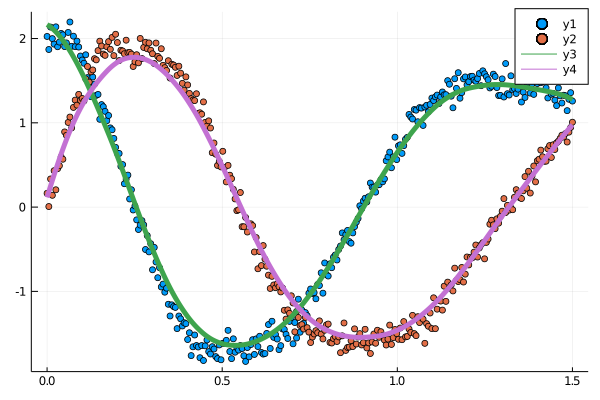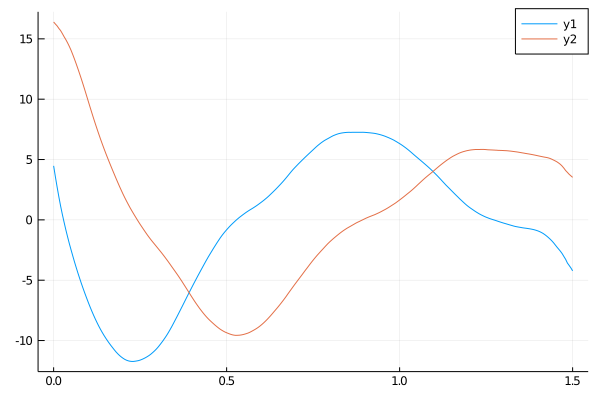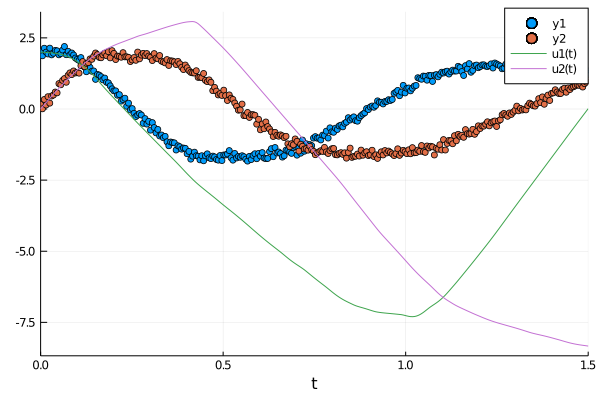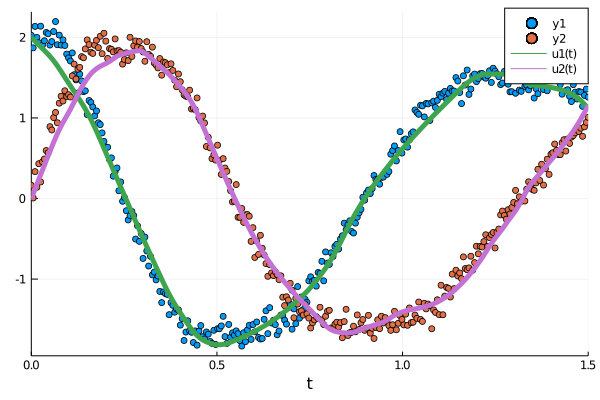# Smoothed Collocation for Fast Two-Stage Training

One can avoid a lot of the computational cost of the ODE solver by pretraining the neural network against a smoothed collocation of the data. First the example and then an explanation.

using Lux, DiffEqFlux, OrdinaryDiffEq, SciMLSensitivity, Optimization, OptimizationFlux, Plots

using Random
rng = Random.default_rng()

u0 = Float32[2.0; 0.0]
datasize = 300
tspan = (0.0f0, 1.5f0)
tsteps = range(tspan, tspan, length = datasize)

function trueODEfunc(du, u, p, t)
true_A = [-0.1 2.0; -2.0 -0.1]
du .= ((u.^3)'true_A)'
end

prob_trueode = ODEProblem(trueODEfunc, u0, tspan)
data = Array(solve(prob_trueode, Tsit5(), saveat = tsteps)) .+ 0.1randn(2,300)

du,u = collocate_data(data,tsteps,EpanechnikovKernel())

scatter(tsteps,data')
plot!(tsteps,u',lw=5)
savefig("colloc.png")
plot(tsteps,du')
savefig("colloc_du.png")

dudt2 = Lux.Chain(x -> x.^3,
Lux.Dense(2, 50, tanh),
Lux.Dense(50, 2))

function loss(p)
cost = zero(first(p))
for i in 1:size(du,2)
_du, _ = dudt2(@view(u[:,i]),p, st)
dui = @view du[:,i]
cost += sum(abs2,dui .- _du)
end
sqrt(cost)
end

pinit, st = Lux.setup(rng, dudt2)

callback = function (p, l)
return false
end

optf = Optimization.OptimizationFunction((x,p) -> loss(x), adtype)
optprob = Optimization.OptimizationProblem(optf, Lux.ComponentArray(pinit))

result_neuralode = Optimization.solve(optprob, ADAM(0.05), callback = callback, maxiters = 10000)

prob_neuralode = NeuralODE(dudt2, tspan, Tsit5(), saveat = tsteps)
nn_sol, st = prob_neuralode(u0, result_neuralode.u, st)
scatter(tsteps,data')
plot!(nn_sol)
savefig("colloc_trained.png")

function predict_neuralode(p)
Array(prob_neuralode(u0, p, st))
end

function loss_neuralode(p)
pred = predict_neuralode(p)
loss = sum(abs2, data .- pred)
return loss
end

optf = Optimization.OptimizationFunction((x, p) -> loss_neuralode(x), adtype)
optprob = Optimization.OptimizationProblem(optf, Lux.ComponentArray(pinit))

numerical_neuralode = Optimization.solve(optprob,
callback = callback,
maxiters = 300)

nn_sol, st = prob_neuralode(u0, numerical_neuralode.u, st)
scatter(tsteps,data')
plot!(nn_sol,lw=5)
savefig("post_trained.png")

## Generating the Collocation

The smoothed collocation is a spline fit of the datapoints which allows us to get a an estimate of the approximate noiseless dynamics:

using Lux, DiffEqFlux, Optimization, OptimizationFlux, DifferentialEquations, Plots

using Random
rng = Random.default_rng()

u0 = Float32[2.0; 0.0]
datasize = 300
tspan = (0.0f0, 1.5f0)
tsteps = range(tspan, tspan, length = datasize)

function trueODEfunc(du, u, p, t)
true_A = [-0.1 2.0; -2.0 -0.1]
du .= ((u.^3)'true_A)'
end

prob_trueode = ODEProblem(trueODEfunc, u0, tspan)
data = Array(solve(prob_trueode, Tsit5(), saveat = tsteps)) .+ 0.1randn(2,300)

du,u = collocate_data(data,tsteps,EpanechnikovKernel())

scatter(tsteps,data')
plot!(tsteps,u',lw=5)We can then differentiate the smoothed function to get estimates of the derivative at each datapoint:

plot(tsteps,du')Because we have (u',u) pairs, we can write a loss function that calculates the squared difference between f(u,p,t) and u' at each point, and find the parameters which minimize this difference:

dudt2 = Lux.Chain(x -> x.^3,
Lux.Dense(2, 50, tanh),
Lux.Dense(50, 2))

function loss(p)
cost = zero(first(p))
for i in 1:size(du,2)
_du, _ = dudt2(@view(u[:,i]),p, st)
dui = @view du[:,i]
cost += sum(abs2,dui .- _du)
end
sqrt(cost)
end

pinit, st = Lux.setup(rng, dudt2)

callback = function (p, l)
return false
end

optf = Optimization.OptimizationFunction((x,p) -> loss(x), adtype)
optprob = Optimization.OptimizationProblem(optf, Lux.ComponentArray(pinit))

result_neuralode = Optimization.solve(optprob, ADAM(0.05), callback = callback, maxiters = 10000)

prob_neuralode = NeuralODE(dudt2, tspan, Tsit5(), saveat = tsteps)
nn_sol, st = prob_neuralode(u0, result_neuralode.u, st)
scatter(tsteps,data')
plot!(nn_sol)While this doesn't look great, it has the characteristics of the full solution all throughout the timeseries, but it does have a drift. We can continue to optimize like this, or we can use this as the initial condition to the next phase of our fitting:

function predict_neuralode(p)
Array(prob_neuralode(u0, p, st))
end

function loss_neuralode(p)
pred = predict_neuralode(p)
loss = sum(abs2, data .- pred)
return loss
end

optf = Optimization.OptimizationFunction((x, p) -> loss_neuralode(x), adtype)
optprob = Optimization.OptimizationProblem(optf, Lux.ComponentArray(pinit))

numerical_neuralode = Optimization.solve(optprob,
plot!(nn_sol,lw=5)